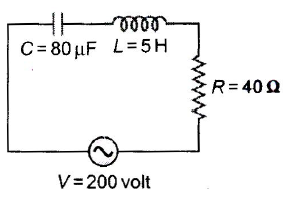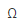Courses

# Alternative Current MCQ Level - 1

## 30 Questions MCQ Test Exclusive Video Lectures of Class 12 Physics by Experts | Alternative Current MCQ Level - 1

Description
This mock test of Alternative Current MCQ Level - 1 for Class 12 helps you for every Class 12 entrance exam. This contains 30 Multiple Choice Questions for Class 12 Alternative Current MCQ Level - 1 (mcq) to study with solutions a complete question bank. The solved questions answers in this Alternative Current MCQ Level - 1 quiz give you a good mix of easy questions and tough questions. Class 12 students definitely take this Alternative Current MCQ Level - 1 exercise for a better result in the exam. You can find other Alternative Current MCQ Level - 1 extra questions, long questions & short questions for Class 12 on EduRev as well by searching above.
QUESTION: 1

Solution:
QUESTION: 2

Solution:
QUESTION: 3

### In a circuit L, C and R are connected in series with an alternating voltage source of frequency f. The current leads the voltage by 45°. The value of C is

Solution:
QUESTION: 4

The natural frequency (ω0) of oscillations in L-C circuit is given by

Solution:
QUESTION: 5

In L-C-R series circuit the resonance condition in terms of capacitive reactance (XC) and inductive reactance (XL) is

Solution:
QUESTION: 6

Average power generated in an inductor connected to an AC source is

Solution:
QUESTION: 7

In L-C-R circuit if resistance increases, quality factor

Solution:
QUESTION: 8

An electric bulb has a rated power of 50 W at 100 V. If it is used on an AC source 200 V, 50 Hz, a choke has to be used in series with it. The should have an inductance of

Solution:
QUESTION: 9

From figure shown below a series L-C-R circuit connected to a variable frequency 200 V source. C = 80μF and R = 40W . Then the source frequency which drive the circuit at resonance isSolution:
QUESTION: 10

In an L-C-R series AC circuit, the voltage across each of components. L,C and R is 50 V. The voltage across the L-C combination will be

Solution:
QUESTION: 11

In an L-C-R circuit, capacitance is changed from C to 2C. For the resonant frequency to remain unchanged, the inductance should be changed from L to

Solution:
QUESTION: 12

Which of the following statement is incorrect?

Solution:
QUESTION: 13

The impedance of a circuit, when a resistance R and an Inductor of inductance L are connected in series in an AC circuit of frequency f, is

Solution:
QUESTION: 14

ln a series L-C-R circuit, resistance R = 10W and the impedance Z = 10W. The phase difference between the current and the voltage is.

Solution:
QUESTION: 15

A circuit consists of an inductance of 0.5 mH and a capacitor of 20μF. The frequency of the L-C oscillations is approximately

Solution:
QUESTION: 16

An inductor is connected to an AC source. When compared to voltage, the current in the lead wires

Solution:
QUESTION: 17

An inductance 1 H is connected in series with an AC source of 220 V and 50 Hz. The inductive reactance (in ohm) is

Solution:
QUESTION: 18

The power factor of an AC circuit having resistance R and inductance L (connected in series) and an angular velocity ω is

Solution:
QUESTION: 19

If the capacity of a condenser is 1 F, then its resistance in a DC circuit will be

Solution:
QUESTION: 20

A capacitor 50μF is connected to a supply of 220 V and angular frequency 50 rad s-1. The value of rms current in the circuit is

Solution:
QUESTION: 21

In a series L-C-R circuit the frequency of a 10 V AC voltage source is adjusted in such a fashion that the reactance of the inductor measures15and that of the capacitor11. If R = 3, the potential difference across the series combination of L and C will be

Solution:
QUESTION: 22

A circuit draws 330 W from a 110 V, 60 Hz AC line. The power factor is 0.6 and the current lags the voltage. The capacitance of a series capacitor that will result in a power factor of unity is equal to

Solution:
QUESTION: 23

In L-R circuit, resistance is 8and inductive reactanceis 6,  then impedance is

Solution:
QUESTION: 24

In L-C-R circuit, an alternating emf of angular frequency ω is applied then the total impedance will be

Solution:
QUESTION: 25

In AC circuit a resistance of Ris connected in series with an inductance L. If the phase difference between the current and voltage is 45°, the inductive reactance will be

Solution:
QUESTION: 26

The current which does not contribute to the power consumed in an AC circuit is called?

Solution:
QUESTION: 27

The power loss in AC circuit will be minimum when

Solution:
QUESTION: 28

In a series combination R = 300, L = 0.9 H, C = 2.0 μF, ω = 1000 rads-1, the impedance of the circuit is

Solution:
QUESTION: 29

For a series L-C-R circuit, the phase difference between current and voltage at the condition of resonance will be

Solution:
QUESTION: 30

In a L-R circuit of 3 mH inductance and 4resistance, emf E = 4 cos 1000tV is applied. The amplitude of emf is

Solution: